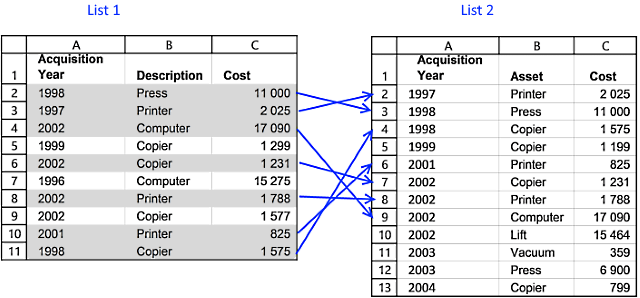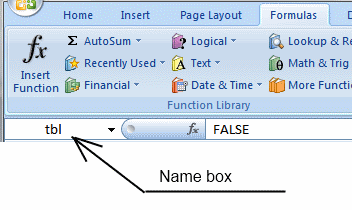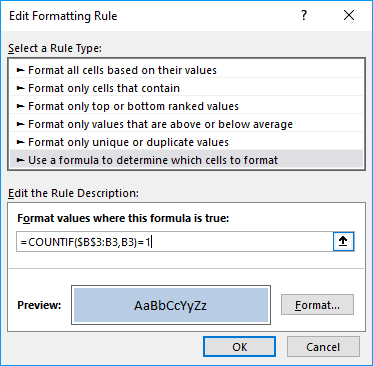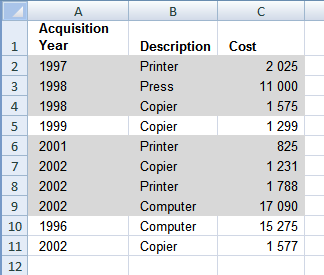Author: Oscar Cronquist Article last updated on January 09, 2019In this blog post I will demonstrate a conditional formatting formula that will highlight common records in two lists. The image above shows you highlighted records in List 1 that also exists in sheet2.

You can also use a formula to extract shared records or an Excel defined table, if you prefer that. There is also an article written for Comparing two columns and highlight values in common.

There are more links to related articles in the sidebar.

### Create named ranges

When constructing a conditional formatting formula you can only reference cells on current sheet. But there is a workaround. The answer is named ranges.

Here is how to create named ranges for this example:

1. Go to sheet List 2
2. Select cell range A2:A13
3. Press with left mouse button on in Name box
4. Type a name (Year)
5. Repeat step 1 to 4 with remaining columns.### Setup COUNTIFS function

The COUNTIFS function looks for duplicate records in the second list (List 2).

COUNTIFS(criteria_range1,criteria1, criteria_range2, criteria2...)
Counts the number of cells specified by a given set of conditions or criteria

In this example:
List 1:Column A - List 2: Column A (Year)
List 1:Column B - List 2: Column B (Asset)
List 1:Column C - List 2: Column C (Cost)

COUNTIFS(YEAR, \$A2, ASSET, \$B2, COST, \$C2)

This formula contains absolute and relative cell references. The conditional formatting formula must look for values in matching columns.

\$A2 is a cell reference to values in column A. The column (\$A) is absolute but the row (2) is relative. When the conditional formatting formula runs the next row, only the row number changes, example \$A3. The named ranges do not change.

If the COUNTIFS function finds one matching record it returns 1, if nothing is found 0 (zero) is returned. 0 (zero) is the equivalent to FALSE so the Conditional formatting will not highlight a cell i FALSE is returned.

### Highlight common records from two lists Excel 2007

How to apply conditional formatting formula:

1. Select cells A2:C11 (Sheet: List 1)
2. Press with left mouse button on "Home" tab
3. Press with left mouse button on "Conditional Formatting" button
4. Press with left mouse button on "New Rule.."
5. Press with left mouse button on "Use a formula to determine which cells to format"
6. Type =COUNTIFS(YEAR, \$A2, ASSET, \$B2, COST, \$C2) in "Format values where this formula is TRUE" window.(The formula shown in the image above is not used in this article)
7. Press with left mouse button on "Format.." button
8. Press with left mouse button on "Fill" tab
9. Select a color for highlighting cells.
10. Press with left mouse button on "Ok"
11. Press with left mouse button on "Ok"
12. Press with left mouse button on "Ok"### Highlight common records from two lists Excel 2003

Conditional formatting formula:

=SUMPRODUCT(COUNTIF(\$A2, Year)*COUNTIF(\$B2, Asset)*COUNTIF(\$C2, Cost))### Get the Excel fileCompare-lists-Highlight-common-values.xlsx